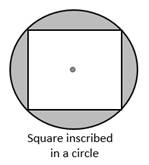Chapter 8.CT, Problem 13CTElementary Geometry For College St...

7th Edition
Alexander + 2 others
ISBN: 9781337614085

Solutions

Chapter
SectionElementary Geometry For College St...

7th Edition
Alexander + 2 others
ISBN: 9781337614085
Textbook Problem

In the figure, a square is inscribed in a circle. If each side of the square measures 4 2 in., find an expression for the exact area of the shaded region.To determine

To find:

An expression for the exact area of the shaded region.

Explanation

Given:

In the fig, a square is inscribed in a circle. If each side of the square measures 42 in.

Theorem:

1. The area A of a circle whose radius has the length r is given by the formula, A=πr2.

2. The area A of the square whose length of the side a is given by A=a2.

From the given, the lengths of the side of the square is 42 in.

Here a=42 and d=a2+a2=2a2=a2, then r=d2=a22=a2

The area of the shaded region = Area of the circle – Area of the square

A=πr2-a2

A=πa22-a2

A=πa22-a2

Still sussing out bartleby?

Check out a sample textbook solution.

See a sample solution

The Solution to Your Study Problems

Bartleby provides explanations to thousands of textbook problems written by our experts, many with advanced degrees!

Get Started

In Exercises 4143, find the distance between the two points. 41. (2, 3) and (1, 7)

Applied Calculus for the Managerial, Life, and Social Sciences: A Brief Approach

Evaluate the expression sin Exercises 116. 52535252

Finite Mathematics and Applied Calculus (MindTap Course List)

In Problems 17-24, find the slope and y-intercept and then graph each equation.

Mathematical Applications for the Management, Life, and Social Sciences